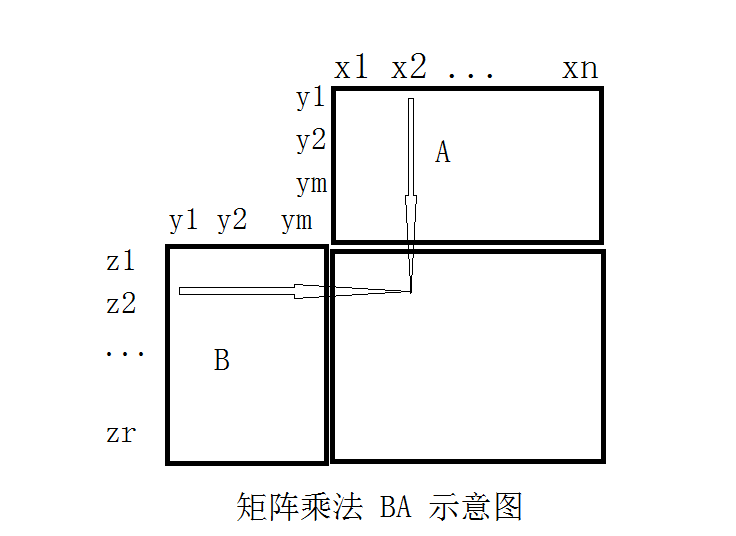# 再谈矩阵与矩阵乘法

《图示矩阵分块乘法》《理解矩阵与矩阵乘积（三）》《理解矩阵与矩阵乘积（二）》《理解矩阵与矩阵乘积（一）》

1                     a11          a12         a13                       a1n
2                     a21          a22         a23                       a2n
…                     …..           ….          ….                         ….
m                    am1         am2         am3                     ann

1                       ?
2

r

c11=a11b11+a21b12+…+am1b1m。# 怎样用一般幂函数的积分公式理解$$y=1/x$$的积分

$(x^a)’=ax^{a-1},\> x>0$

$\frac{(x^a)’}{a}=x^{a-1}$

$\frac{\partial _x x^y}{y}=x^{y-1}$

$\frac{\partial_x x^y-\partial_x x^0}{y}=x^{y-1}$

$\left. \partial_y(\partial_x x^y)\right |_{y=0}=x^{-1}$

$\partial_x(\left. \partial_y x^y\right|_{y=0})=x^{-1}$

$(\ln x)’=\frac{1}{x}$

$\partial_y(\partial_x x^y)=x^{y-1}+yx^{y-1}\ln x$

# 用定积分的定义计算双曲线下方图形的面积

http://tieba.baidu.com/p/3475129628

1) 在双曲线上任意一点向两坐标轴做垂线，证明这两条垂线与坐标轴形成的矩形的面积与点的位置无关，这个面积是多少？
2) 用上述特性在双曲线与坐标轴之间做出无穷多个互不重叠的矩形，并且每个矩形的面积不小于一个定值，比如$$0.9$$。（提示：先做出一个面积为1的正方形，再做出一个 1）中所描述的矩形，二者重叠部分的面积有什么特点？）

3) 将 2) 中的矩形面积不断减小，比如，让所有矩形的面积都等于$$\epsilon$$（那个正方形除外），这些矩形的宽度就会不断减小，\)[1,t]\)之间的曲边梯形就会逐渐被一些面积相等的矩形所铺满。计算\)[1,t]\)之间矩形的个数，并用\)\epsilon\)表示。
4）计算这些矩形的总面积，并计算当$$\epsilon\to 0$$时的极限。证明这些小矩形的宽度随着$$\epsilon\to 0$$而趋于零。根据反比例函数在$$[1,t]$$上的可积性，这些矩形总面积的极限就是曲边梯形的面积。

5）为了比较$$y=1/x$$与$$y=1/x^2$$的差别，把这套策略改造一下应用到函数$$y=1/x^2$$上，并解释为什么对数函数没有出现在$$y=1/x^2$$的下方。

# 用超限归纳法证明《实变函数论》中另一命题

《实变函数论》中在证明了这个定义的合理性（即 E 的函数值与 E 的初等分解方式无关）之后，作者通过134页（2009清华版124页）引理2.3.2 证明了这个集合函数的一系列性质，包括有限可加性，单调性和有限次可加性，最后证明这个集合函数是环 $$\mathscr R_0$$ 上的测度，即满足空集的函数值为0；此函数非负，且具有可数可加性。